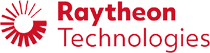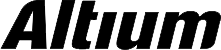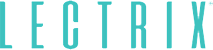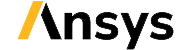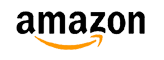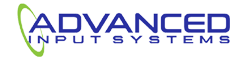﻿ Kelly Criterion Explained | NWES Blog## Kelly Criterion Explained

By ZM Peterson • Jun 16, 2019

I often receive questions about the Kelly criterion and how to develop management models around it. The simple answer is that Kelly’s criterion is one way to reconcile risk and reward associated with financial decisions, allowing one to calculate average returns on investment over a sufficiently large period of time. While the original incarnation of the Kelly criterion actually had nothing to do with financial modeling or portfolio management, it has found a solid home in this mathematically rich area.

Rather than repeat all of my results from my recent paper in Journal of Investment Strategies, I’ll take some time to summarize the core concepts in the Kelly criterion so that interested readers have a baseline for further study.

## Return vs. Risk in the Kelly Criterion

Anyone with a passing knowledge of portfolio management, the capital asset pricing model (CAPM), and other financial models for predicting returns on investment knows that risk and reward are inherently scaled. With loans, a riskier borrower should rationally pay a larger interest rate, with the goal being to earn a profit on the loan before the borrower defaults. With a new company, investors should expect to see a larger return when investing in riskier enterprises in exchange for investors' tolerance of a potential loss on their investment. The same ideas apply to stocks, cryptocurrencies, or really any security whose value fluctuates over time.

The goal in any portfolio management problem is to balance risk and return. There are several ways to quantify the tradeoff between risk and return. One popular metric is to use the Sharpe ratio, which measures the return in excess of the return on risk-free assets, divided by the volatility (standard deviation) in the asset’s value. These measures are taken over somewhat arbitrarily chosen time periods, although there are a number of techniques for examining how this and other metrics change over time.

Portfolio management problems quantify risk and return as follows:

• Return is taken as the expected value of changes in the value of assets in the portfolio. Again, this expected return is taken over a particular time period.
• Risk is taken as the covariance among the value of various assets in the portfolio. In other words, this measures the fluctuation in the value of assets in the portfolio over time, as well as how asset values move together over time.

In models based on the Kelly criterion, one can derive an asymptotic equation for the average return on a portfolio of assets over time. As time goes on to infinity, and under a reasonable rebalancing strategy, one can calculate how the return on investment will behave and eventually approach a specific value.

One should note that, in CAPM, risk on a specific asset is actually taken as the covariance between each asset in the portfolio and the rest of the market. Risk in the standard Markowitz model and in models based on the Kelly criterion do not consider the rest of the market, and one can show mathematically that correlations with the market should not appear in the risk function. However, one still cannot discount the influence of market forces on groups of correlated assets when analyzing the behavior of assets in the market. Therefore, some incarnations of portfolio management models consider the beta for assets in the portfolio instead of their intrinsic volatility.

## Optimized Returns

If one examines the behavior of the value of the portfolio, and thus the returns, one finds that there is a specific fraction of wealth that one should invest in each asset that will maximize the return on investment. This idea is absent from the function defining returns under the Markowitz model, which simply looks at a weighted sum of the asset values in the portfolio. If risk is ignored in the Markowitz model, the logical choice would be to allocate the largest possible amount of money (100% of funds) to the asset with the highest expected return.

To see why this is a bad idea, let’s consider bets in a dice game. Suppose you are playing a dice game where a roll of 1 means you lose your bet, rolls of 2 to 5 mean you win and lose nothing, and a roll of 6 triples your money. The expected return from this dice game is If you were to bet 0% of your total capital on every dice game, your return would be 0%. According to Markowitz, your return per bet is linearly proportional to your bet, and it would be 8.33% per bet if you wagered 100% of your money.

Under a single bet, this is fine, although you still risk losing all of your capital. The primary difference under the Kelly criterion is this: The Kelly criterion looks at what happens over a large number of bets.

If you bet 100% of your money in every single round of this simple dice game, the probability of losing your money approaches 1. This consideration is absent from the Markowitz model. If you were to bet 0% of your money in every dice game, then your return is 0%. Obviously, there is some amount of capital (between 0% and 100%) that will yield the maximum return on investment. The goal in the Kelly criterion is to determine this ideal fraction of capital that maximizes your return.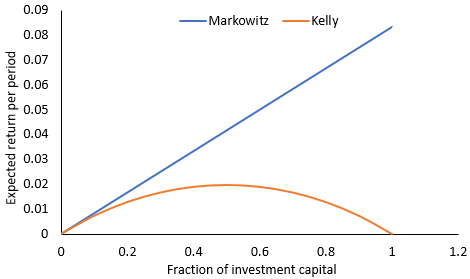Returns on a single bet according to Kelly and Markowitz

Typically, portfolio management involving the Kelly criterion is framed as an optimization problem with a goal of maximizing the average return one would expect to see over successive time periods. Conceptually, this aligns the Kelly criterion with the Markowitz problem, although the results are quite different. An example with a single asset is shown in the graph above. Note that, according to Markowitz while ignoring of risk, the optimized return occurs when the fraction of invested capital is 100% during each period. However, there is a sweet spot with the Kelly criterion, where the optimal fraction of capital to invest is approximately 50%.

## Multiple Assets

The Kelly criterion can be extended to treat multiple assets, and the expected return on the portfolio can be derived directly from a stochastic process for the portfolio. In this case, the expected return is: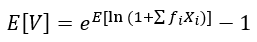Returns according to the Kelly criterion for multiple assets

Computing the above expected value requires knowledge of the joint probability distribution for the portfolio. Note that, for a broad range of distributions (including a joint normal distribution), the above equation does not have an analytical solution. This is where a variety of numerical techniques or approximations become critical to calculating the average return for the Kelly criterion. The author provides a similar formulation that does not require use of the joint probability distribution for the assets in the portfolio. You can find this article in Journal of Investment Strategies.

When examining the tradeoff between risk and return for a portfolio of multiple assets, one can generate a Pareto front that illustrates the tradeoff between risk and return. An example is shown below. The values of risk and return can then be analyzed using any standard financial index one likes, such as the Sharpe index or the Treynor index.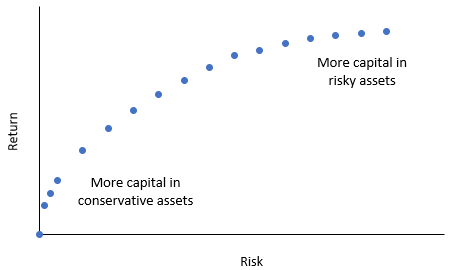Example Pareto front for a portfolio

These articles are excellent references for novice readers that are interested in working with the Kelly criterion and its extensions into more complicated areas of analysis.

• Kelly Jr, J. L. A new interpretation of information rate. In The Kelly Capital Growth Investment Criterion: Theory and Practice (pp. 25-34), 2011.
• Peterson, Z. Kelly's Criterion in Portfolio Optimization: A Decoupled Problem. Journal of Investment Strategies 7(2), 2018.
• Rotando, L. M., & Thorp, E. The Kelly Criterion and the Stock Market. The American Mathematical Monthly 99(10):922-931, 1992.
• Browne, S., & Whitt, W. Portfolio Choice and the Bayesian Kelly Criterion. Advances in Applied Probability 28(4):1145-1176, 1996.
• Hsieh, C.-H., Gubner, J. A., & Barmish, B. R. Rebalancing Frequency Considerations for Kelly-Optimal Stock Portfolios in a Control-Theoretic Framework. 2018 IEEE Conference on Decision and Control (CDC), Miami Beach, FL, USA, Dec. 17-19, 2018
• Byrnes, T., & Barnett, T. Generalized framework for applying the Kelly criterion to stock markets. International Journal of Theoretical and Applied Finance 21(05):1850033, 2015.

* indicates required

## Our Clients and Partners